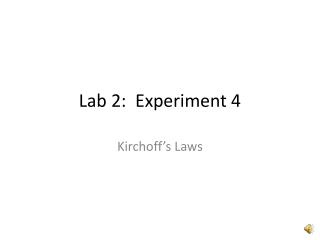# Lab 2: Experiment 4 - PowerPoint PPT PresentationDownload PresentationLab 2: Experiment 4

Lab 2: Experiment 4
Download Presentation## Lab 2: Experiment 4

- - - - - - - - - - - - - - - - - - - - - - - - - - - E N D - - - - - - - - - - - - - - - - - - - - - - - - - - -
##### Presentation Transcript

1. Lab 2: Experiment 4 Kirchoff’s Laws

2. Circuits to be Constructed Circuit from Lab Manual Circuit from Instructions

3. Trim Potentiometers • Trim pots, for short • A resistor whose values depends on the position of the wiper (middle terminal – Pin 2). • Used as a voltage divider • All three terminals are connected in the circuit. • Used as a variable resistor • Either pins 1 and 2 or 2 and 3 are connected in the circuit.

4. Pinout • Pins 1 and 3 are labeled on the top surface of the trim pot. • The resistance between pins 1 and 3 is the maximum resistance of the trim pot (Rpot). • The middle pin (2) is connected to the wiper. • The resistance between pins 1 and 2 is x Rpot, where x is the fraction of the total number of turns of the knob. • The resistance between pins 2 and 3 is (1 – x) Rpot, where x is the fraction of the total number of turns of the knob. • There may be a notation on the top surface about the direction that the knob should be turned [Clockwise (CW) or Counterclockwise (CCW)] to increase the value of the resistance between pins 1 and 2 and decrease the value of the resistance between pins 2 and 3. http://www.solarbotics.com/assets/images/rt1k_t/rt10k-t-dscn3762_pl.JPG

5. Reading the Value of Your Trim Pot • On one surface of the pot are markings • The maximum resistance of the trim pot • The part number • The value of the resistance is calculated as follows: • The first two digits of the three digit number is the number that is then multiplied by 10 raised to the third digit. • For example: 102 = 10 x 102 = 1 kW

6. Pspice Symbol POT: Trim Pot R_Var: Variable Resistor

7. PSpice Simulations Bias Point and DC Sweeps

8. Bias Point • Single calculation of the steady-state currents and voltages in the circuit. • If only d.c. sources are present, then the values are the d.c. currents and voltages. • If only a.c. sinusoidal sources are present, then the values are the average a.c. currents and voltages (i.e., 0A and 0V since the average of sinusoid is zero).

9. DC Sweep • Calculations of voltages and currents as the value of one or more components are varied. • The component can be a voltage or current source, a resistor, a parameter within a device model, or the ambient temperature, which causes changes in all temperature sensitive components. • Plots of voltages and currents as a function of the changing value of the component are automatically made when voltage or current markers are placed on the schematic.